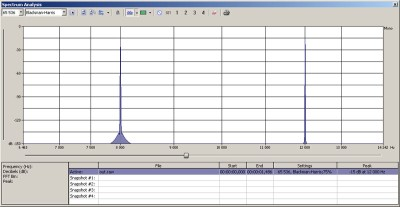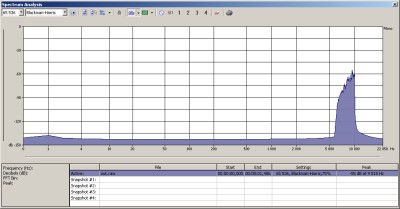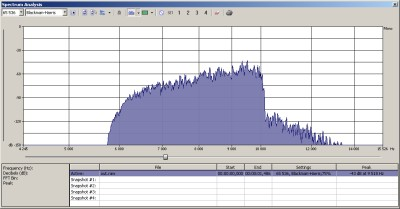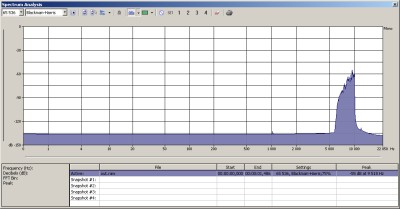### SSB modulation in software

NOTE: this post is outdated. See my ham DSP tutorial!

Single-sideband is one of the most popular modulation modes in analog voice radio communication today. SSB avoids the bandwidth doubling and unnecessary carrier emitting of amplitude modulation, thus concentrating radiated power only on the signal that carries the important information (for ex. voice).

If we take a 2KHz sine wave (modulator wave, this is the information we want to transport, so after demodulation, we would like to get this 2KHz beep) and modulate a 10KHz sine wave (carrier wave, this will carry our modulator wave) with it (you simply multiply the two sines), you get this:You can see the two sidebands: LSB – lower sideband, the 8KHz sine, USB – upper sideband, the 12KHz sine. If you do this 2 sine multiplication in analog electronics, you will also get the 10KHz carrier wave.

Now this means if you want to transmit this 2KHz sine wave over radio on (for example) 3.791MHz, in theory you multiply the 2KHz sine with a 3.791MHz sine and transmit that resulting signal. In this case you will have to transmit both sidebands – if you transmit with 100 watts, your output power will distribute between the two sidebands, so this is not good, you only want to transmit one of the sidebands with full power.A 4KHz wide LSB modulated voice signal

I played with three methods of generating an SSB modulated signal in software.

The first method is a very straight approach and only can be realized in software with no real benefit: let’s multiply our modulator wave with the carrier and then lowpass (or highpass) the sideband we want to keep.

This needs very sharp filtering. In the example I used FFT on the output, zeroed out all the unnecessary bins and then made an inverse FFT and got the result.

 123456789101112131415161718192021222324252627282930313233343536373839 void CssbtestDlg::FillBuffer( float* pBuffer, int nBufferLength ) {     int fft_i = 0;     for( int i = 0; i < nBufferLength; i++ )     {         pBuffer[i] = (float)(0.5 * sin( 2*M_PI * 200 * i / (float)SAMPLESPERSECOND ) ) +             + (float)(0.5 * sin( 2*M_PI * 500 * i / (float)SAMPLESPERSECOND ) ) +             (float)(0.5 * sin( 2*M_PI * 1000 * i / (float)SAMPLESPERSECOND ) ) +             (float)(0.5 * sin( 2*M_PI * 2000 * i / (float)SAMPLESPERSECOND ) );         pBuffer[i] *= (float)sin( 2*M_PI * (10000) * i / (float)SAMPLESPERSECOND ); // osc1         // filter         if( fft_i++ == FFT_RES-1 )         {             for( int j=0; j < FFT_RES; j++ )             {                 fft_in[j] = pBuffer[i-(FFT_RES-1)+j];                 fft_in[j] = 0;             }             fftw_execute(fft_pf);             for( int j=10000; j < FFT_RES; j++ )                 fft_out[j] = fft_out[j] = 0;             fftw_execute(fft_pb);             for( int j=0; j < FFT_RES; j++ )                 pBuffer[i-(FFT_RES-1)+j] = fft_in[j]/FFT_RES;             fft_i = 0;         }     }     // demod     //for( int i = 0; i < nBufferLength; i++ )     //  pBuffer[i] *= (float)sin( 2*M_PI * 10000 * i / (float)SAMPLESPERSECOND ); }

Our buffer is exactly 44100 bytes long (this is the samplerate I used) and I set FFT_RES to this too, so the FFT library’s (libfftw) output is exactly linear, the 10000th bin means 10KHz and so on. In the example my carrier wave is a 10KHz sine, so I zeroed out all frequency components above that to get the lower sideband.

For demodulation of an SSB signal you can multiply it with a sine which has the frequency of the carrier wave (in this example 10KHz).

The second method is called the Hartley method. This method can be simulated very efficiently and easily in software. To generate an SSB signal with this method, two versions of the original signal are generated, mutually 90Â° out of phase. Each one of these signals is then mixed with carrier waves that are also 90Â° out of phase with each other. By either adding or subtracting the resulting signals, a lower or upper sideband signal results.

90 degrees phase delay can be realized with a Hilbert transformer. You can design one using this great page.

 1234567891011121314151617181920212223242526 #define NZEROS 512 #define GAIN   1.570483967e+00//out = (float)(0.2 * sin( 2*M_PI * 200 * i / (float)SAMPLESPERSECOND ) ); // SINE         //out += (float)(0.2 * (float)sin( 2*M_PI * 500 * i / (float)SAMPLESPERSECOND ) ); // SINE         //out += (float)(0.2 * (float)sin( 2*M_PI * 800 * i / (float)SAMPLESPERSECOND ) ); // SINE         for( j = 0; j < NZEROS; j++ )             xv[j] = xv[j+1];             xv[NZEROS] = (float)(out / GAIN);             sum = 0.0;         for( j = 0; j <= NZEROS; j++)             sum += (float)( xcoeffs[j] * xv[j] );         for( j = 0; j < NZEROS/2; j++ )             delay[j] = delay[j+1];             delay[NZEROS/2] = out;         pBuffer[i] = delay   *   cos( 2*M_PI * 10000 * i / (float)SAMPLESPERSECOND ) +             sum   *   sin( 2*M_PI * 10000 * i / (float)SAMPLESPERSECOND );         // demod         //pBuffer[i] *= (float)sin( 2*M_PI * 10000 * i / (float)SAMPLESPERSECOND );         voicep++;         if( voicep == 120040 )             voicep = 0;     } }

In this example I used a prerecorded voice with 4KHz bandwidth as the modulator. The carrier is at 10KHz. The resulting LSB signal can be seen here:The third method is called the Weaver method. This is the most complicated one, it uses two quadrature oscillators and lowpass filters. A very nicely illustrated paper on this method can be downloaded here, read this if you want to learn the mechanisms of this method.

For lowpass filtering I used the previously described FFT – inverse FFT method.

 12345678910111213141516171819202122232425262728293031323334353637383940414243444546474849505152535455565758596061626364656667686970717273 void CssbtestDlg::FillBuffer( float* pBuffer, int nBufferLength ) {     float pOut1[SAMPLESPERSECOND], pOut2[SAMPLESPERSECOND];     int fft_i = 0;     for( int i = 0; i < nBufferLength; i++ )     {         pOut1[i] = pOut2[i] = voice[voicep];         /*pOut1[i] = pOut2[i] = (float)(0.5 * sin( 2*M_PI * 200 * i / (float)SAMPLESPERSECOND ) ) +             + (float)(0.5 * sin( 2*M_PI * 500 * i / (float)SAMPLESPERSECOND ) ) +             (float)(0.5 * sin( 2*M_PI * 1000 * i / (float)SAMPLESPERSECOND ) ) +             (float)(0.5 * sin( 2*M_PI * 2000 * i / (float)SAMPLESPERSECOND ) );*/         pOut1[i] *= (float)cos( 2*M_PI * (SAMPLESPERSECOND/4) * i / (float)SAMPLESPERSECOND ); // osc1         pOut2[i] *= (float)sin( 2*M_PI * (SAMPLESPERSECOND/4) * i / (float)SAMPLESPERSECOND ); // osc1         // filter         if( fft_i++ == FFT_RES-1 )         {             // filter1             for( int j=0; j < FFT_RES; j++ )             {                 fft_in[j] = pOut1[i-(FFT_RES-1)+j];                 fft_in[j] = 0;             }             fftw_execute(fft_pf);             for( int j=FFT_RES/4; j < FFT_RES; j++ )                 fft_out[j] = fft_out[j] = 0;             fftw_execute(fft_pb);             for( int j=0; j < FFT_RES; j++ )             {                 pOut1[i-(FFT_RES-1)+j] = fft_in[j]/FFT_RES;                 pOut1[i-(FFT_RES-1)+j] *= (float)cos( 2*M_PI * (SAMPLESPERSECOND/4-10000) * (i-(FFT_RES-1)+j) / (float)SAMPLESPERSECOND ); // osc2             }             // filter2             for( int j=0; j < FFT_RES; j++ )             {                 fft_in[j] = pOut2[i-(FFT_RES-1)+j];                 fft_in[j] = 0;             }             fftw_execute(fft_pf);             for( int j=FFT_RES/4; j < FFT_RES; j++ )                 fft_out[j] = fft_out[j] = 0;             fftw_execute(fft_pb);             for( int j=0; j < FFT_RES; j++ )             {                 pOut2[i-(FFT_RES-1)+j] = fft_in[j]/FFT_RES;                 pOut2[i-(FFT_RES-1)+j] *= (float)sin( 2*M_PI * (SAMPLESPERSECOND/4-10000) * (i-(FFT_RES-1)+j) / (float)SAMPLESPERSECOND ); // osc2             }             fft_i = 0;             for( int j=0; j < FFT_RES; j++ )                 pBuffer[i-(FFT_RES-1)+j] = pOut1[i-(FFT_RES-1)+j] + pOut2[i-(FFT_RES-1)+j];         }         voicep++;         if( voicep == 120040 )             voicep = 0;     }     // demod     //for( int i = 0; i < nBufferLength; i++ )     //  pBuffer[i] *= (float)sin( 2*M_PI * 10000 * i / (float)SAMPLESPERSECOND ); }

The resulting signal:I hope you find this post useful. I had great time experimenting with all these stuff. More writings like this will come soon.Marxy 2012-04-21 12:00:15

Wow!

Thanks so much for sharing your code.

I’m wondering if it is possible to generate ssb in real time on an arm processor such as the raspberry pi?

Marxy2012-04-21 12:41:13

I’m sure you don’t need a Raspberry Pi, software SSB modulation will work fine on a much slower embedded PC or MCU.DAVID K OSBURN2013-09-03 18:17:20

Hi, I’m a real beginner, what code is your AM SSB and do you have any suggestions on how I can get started understanding and using the AM SSB up converter software.

Thanks.

David2013-09-03 18:36:04

Hello, you should read my tutorials from the beginning ;)2022-04-02 19:36:542022-04-02 19:35:52

working on SSB in software now :) thanks for your info!

Name (required)
E-mail (required - never shown publicly)
Webpage URL
Comment:
You may use <a href="" title=""> <abbr title=""> <acronym title=""> <b> <blockquote cite=""> <cite> <code> <del datetime=""> <em> <i> <q cite=""> <s> <strike> <strong> in your comment.